Author: Oscar Cronquist Article last updated on March 17, 2021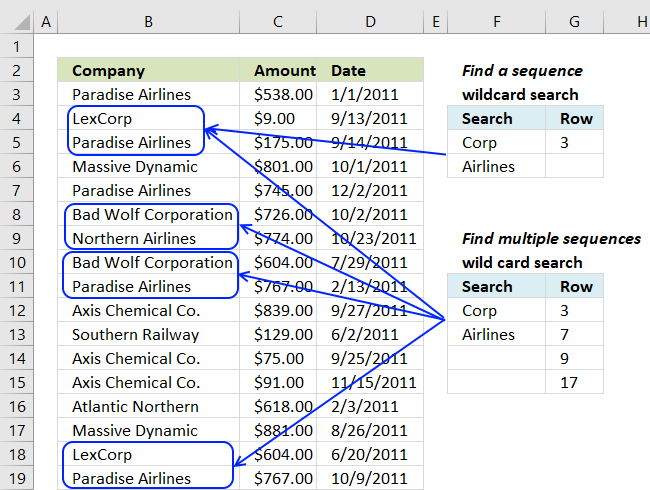This article demonstrates array formulas that perform a wildcard search based on a sequence of values. The formulas return the row number of cells that contain the search values in a consecutive order.

In my last article, I showed you how to find a sequence of values. The array formula extracted the row of the first found sequence.

## 1. How to calculate the first row number of two cells containing search values next to each other vertically?

The array formula in cell F3 returns the row number of the first two cells that contain the given search values.

Array formula in cell F3:

=MATCH(1, COUNTIFS(E3, B1:B23, E4, B2:B24), 0)

### How to enter an array formula

To enter an array formula, type the formula in a cell then press and hold CTRL + SHIFT simultaneously, now press Enter once. Release all keys.

The formula bar now shows the formula with a beginning and ending curly bracket telling you that you entered the formula successfully. Don't enter the curly brackets yourself.

Back to top

### Explaining formula in cell F3

#### Step 1 - Create an array that shows where the specific sequence is

The COUNTIFS function lets you count values based on multiple conditions, in this case, I am also changing the cell references.

The first two arguments check for the value in cell E3 in cell range B1:B23. The third and fourth argument looks for the value in cell E4 in cell range B2:B24, note that the cell range in argument 4 relative the 2nd argument is offset by 1.

This cell reference technique lets you find values in a given sequence.

COUNTIFS(E3,B1:B23,E4,B2:B24) returns {0; 0; 0; 0; 0; 0; 0; 0; 1; 0; 0; 0; 0; 0; 0; 0; 0; 1; 0; 0; 0; 0; 0}.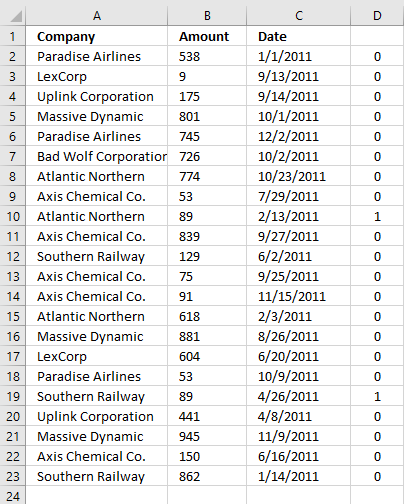The array is shown in column D.

#### Step 2 - Find the relative position in the array

The MATCH function finds the position in the array based on a given value, if multiple values exist the position of the first is returned.

MATCH(1, COUNTIFS(E3, B1:B23, E4, B2:B24), 0)

becomes

MATCH(1, {0; 0; 0; 0; 0; 0; 0; 0; 1; 0; 0; 0; 0; 0; 0; 0; 0; 1; 0; 0; 0; 0; 0}, 0)

and returns 9 in cell F3.

Back to top

## 2. How to calculate all row numbers of two cells containing search values next to each other vertically?

What I didn't show you was how to find multiple sequences with this particular array formula.This array formula finds all instances of cells containing a sequence of values, the order is important. It returns their starting row number.

Array formula in cell F8:

=SMALL(IF(COUNTIFS(\$E\$8, \$B\$1:\$B\$23, \$E\$9, \$B\$2:\$B\$24)=1, MATCH(ROW(\$A\$1:\$A\$23), ROW(\$A\$1:\$A\$23)), ""), ROW(A1))

How to enter an array formula

Update 3-17-2021, the following Excel 365 formula extracts the corresponding row numbers from the found sequences:

=FILTER(ROW(A2:A23), (E8=B2:B23)*(E9=B3:B24))

Back to top

### Explaining formula in cell F8

See step 1 in the explanation above before reading the rest below.

#### Step 2 - Convert boolean value TRUE to row number in the array

The IF function uses a logical expression in the first argument to determine if the second or third argument is being returned. The second argument if the logical expression evaluates to TRUE and the third argument if the logical expression evaluates to FALSE.

IF(COUNTIFS(\$E\$8, \$B\$1:\$B\$23, \$E\$9, \$B\$2:\$B\$24)=1, MATCH(ROW(\$A\$1:\$A\$23), ROW(\$A\$1:\$A\$23)), "")

becomes

IF({0; 0; 0; 0; 0; 0; 0; 0; 1; 0; 0; 0; 0; 0; 0; 0; 0; 1; 0; 0; 0; 0; 0}, MATCH(ROW(\$A\$1:\$A\$23), ROW(\$A\$1:\$A\$23)), "")

becomes

IF({0; 0; 0; 0; 0; 0; 0; 0; 1; 0; 0; 0; 0; 0; 0; 0; 0; 1; 0; 0; 0; 0; 0}, {1; 2; 3; 4; 5; 6; 7; 8; 9; 10; 11; 12; 13; 14; 15; 16; 17; 18; 19; 20; 21; 22; 23}, "")

and returns

{"";"";"";"";"";"";"";"";9;"";"";"";"";"";"";"";"";18;"";"";"";"";""}

#### Step 3 - Extract row numbers sorted smallest to largest

The SMALL function extracts the k-th smallest number in array, it ignores blanks.

SMALL(IF(COUNTIFS(\$E\$8, \$B\$1:\$B\$23, \$E\$9, \$B\$2:\$B\$24)=1, MATCH(ROW(\$A\$1:\$A\$23), ROW(\$A\$1:\$A\$23)), ""), ROW(A1))

becomes

SMALL({"";"";"";"";"";"";"";"";9;"";"";"";"";"";"";"";"";18;"";"";"";"";""}, ROW(A1))

The ROW function returns the row number based on a cell reference, this cell reference is relative meaning it will change as the formula is copied to cells below. This makes the formula extracting a new value in each cell.

SMALL({"";"";"";"";"";"";"";"";9;"";"";"";"";"";"";"";"";18;"";"";"";"";""}, ROW(A1))

becomes

SMALL({"";"";"";"";"";"";"";"";9;"";"";"";"";"";"";"";"";18;"";"";"";"";""}, 1)

returns 9 in cell F8.

Back to top

## 3. Lookup for a multi-level sequence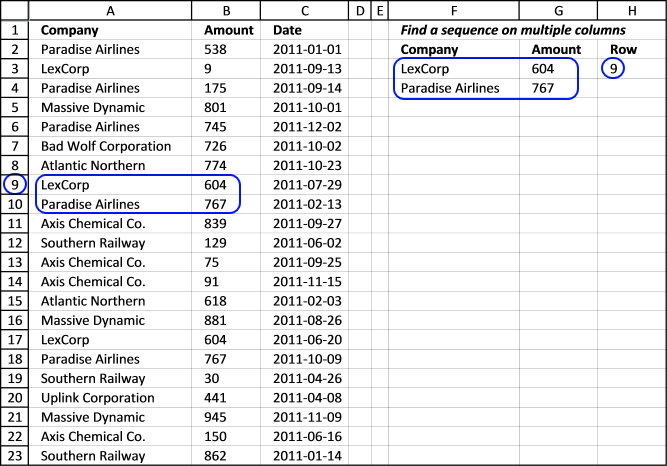The next array formula looks for consecutive values in columns and rows. This means that the values in cell range F3:G4 must be found in column A and B in order to return the corresponding row number.

Array formula in cell H3:

=MATCH(1,COUNTIFS(F3, A1:A23, F4, A2:A24, G3, B1:B23, G4,B2:B24), 0)

Use this formula if you are looking for the first instance of a sequence. It looks for a specific sequence (LexCorp and Paradise Airlines) in column A and for 604 and 767 in column B. LexCorp and 604 must be on the same row and Paradise Airlines and 767 must be on the next row below.

How to enter an array formula

Update 3-17-2021, the following Excel 365 formula extracts the corresponding dates in column C from the found sequences:

=FILTER(C2:C23,(F3=A2:A23)*(G3=B2:B23)*(F4=A3:A24)*(G4=B3:B24))

Back to top

### Explaining formula in cell H3

#### Step 1 - Create an array that shows where the specific sequence is

The COUNTIFS function lets you count values based on multiple conditions, in this case, I am also doing changes to the cell references.

COUNTIFS(F3, A1:A23, F4,A2:A24, G3, B1:B23, G4, B2:B24) returns {0; 0; 0; 0; 0; 0; 0; 0; 1; 0; 0; 0; 0; 0; 0; 0; 1; 0; 0; 0; 0; 0; 0}.

The second record (cell F4 and G4) are offset 1 row in order to find two consecutive records.

#### Step 2 - Find the relative position in the array

The MATCH function finds the position in the array based on a given value if multiple values exist the position of the first is returned.

MATCH(1, COUNTIFS(F3, A1:A23, F4,A2:A24, G3, B1:B23, G4, B2:B24), 0)

becomes

MATCH(1, {0; 0; 0; 0; 0; 0; 0; 0; 1; 0; 0; 0; 0; 0; 0; 0; 1; 0; 0; 0; 0; 0; 0}, 0)

and returns 9 in cell H3.

Back to top

## 4. Lookup for multiple multi-level sequences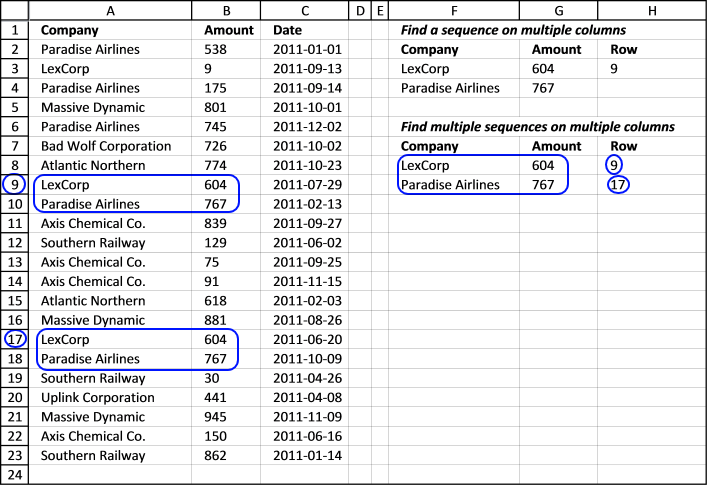Array formula in cell H8:

=SMALL(IF(COUNTIFS(\$F\$8, \$A\$1:\$A\$23, \$F\$9, \$A\$2:\$A\$24, \$G\$8, \$B\$1:\$B\$23, \$G\$9, \$B\$2:\$B\$24)=1, MATCH(ROW(\$A\$1:\$A\$23), ROW(\$A\$1:\$A\$23)), ""), ROW(A1))

It is like the formula above but it returns the location of multiple sequences.

How to enter an array formula

Update 3-17-2021, the following Excel 365 formula extracts the corresponding dates in column C from the found sequences:

=FILTER(ROW(C2:C23),(F8=A2:A23)*(G8=B2:B23)*(F9=A3:A24)*(G9=B3:B24))

Back to top

### Explaining formula in cell H8

See step 1 in the explanation above before reading the rest below.

#### Step 2 - Convert boolean value TRUE to row number in array

The IF function uses a logical expression in the first argument to determine if the second or third argument is being returned. The second argument if the logical expression evaluates to TRUE and the third argument if the logical expression evaluates to FALSE.

IF(COUNTIFS(\$F\$8, \$A\$1:\$A\$23, \$F\$9, \$A\$2:\$A\$24, \$G\$8, \$B\$1:\$B\$23, \$G\$9, \$B\$2:\$B\$24)=1, MATCH(ROW(\$A\$1:\$A\$23), ROW(\$A\$1:\$A\$23)), "")

becomes

IF({FALSE; FALSE; FALSE; FALSE; FALSE; FALSE; FALSE; FALSE; TRUE; FALSE; FALSE; FALSE; FALSE; FALSE; FALSE; FALSE; TRUE; FALSE; FALSE; FALSE; FALSE; FALSE; FALSE}, MATCH(ROW(\$A\$1:\$A\$23), ROW(\$A\$1:\$A\$23)), "")

becomes

IF({FALSE; FALSE; FALSE; FALSE; FALSE; FALSE; FALSE; FALSE; TRUE; FALSE; FALSE; FALSE; FALSE; FALSE; FALSE; FALSE; TRUE; FALSE; FALSE; FALSE; FALSE; FALSE; FALSE}, {1; 2; 3; 4; 5; 6; 7; 8; 9; 10; 11; 12; 13; 14; 15; 16; 17; 18; 19; 20; 21; 22; 23}, "")

and returns

{"";"";"";"";"";"";"";"";9;"";"";"";"";"";"";"";"";17;"";"";"";"";""}.

#### Step 3 - Extract row numbers sorted smallest to largest

The SMALL function extracts the k-th smallest number in array, it ignores blanks.

SMALL(IF(COUNTIFS(\$F\$8, \$A\$1:\$A\$23, \$F\$9, \$A\$2:\$A\$24, \$G\$8, \$B\$1:\$B\$23, \$G\$9, \$B\$2:\$B\$24)=1, MATCH(ROW(\$A\$1:\$A\$23), ROW(\$A\$1:\$A\$23)), ""), ROW(A1))

becomes

SMALL({"";"";"";"";"";"";"";"";9;"";"";"";"";"";"";"";17;"";"";"";"";"";""}, ROW(A1))

The ROW function returns the row number based on a cell reference, this cell reference is relative meaning it will change as the formula is copied to cells below. This makes the formula extracting a new value in each cell.

SMALL({"";"";"";"";"";"";"";"";9;"";"";"";"";"";"";"";17;"";"";"";"";"";""}, ROW(A1))

becomes

SMALL({"";"";"";"";"";"";"";"";9;"";"";"";"";"";"";"";17;"";"";"";"";"";""}, 1)

returns 9 in cell F8.

Back to top

## 5. Wild card search for a sequenceThis demonstrates how to do a wild card search for a sequence. This formula returns the row number of the first found sequence in column A. The formula looks for a text string in a cell and another text string in the cell below. You don't need to enter the wild cards * (asterisks), see cell F3 and F4.

=MATCH(TRUE, ISNUMBER(FIND(\$F\$11, \$A\$1:\$A\$23)*FIND(\$F\$12, \$A\$2:\$A\$24)), 0)

How to enter an array formula

Update 3-17-2021, the following Excel 365 formula extracts the corresponding cell values in column B and C from all found sequences or consecutive values:

=FILTER(C2:D23, ISNUMBER(FIND(\$F\$3, \$A\$2:\$A\$23)*FIND(\$F\$4, \$A\$3:\$A\$24)))

Back to top

### Explaining formula in cell G3

#### Step 1 - Find cells containing search value in cell F11

The FIND function returns the position of a specific string in another string, reading left to right. Note, the FIND function is case-sensitive.

FIND(\$F\$11, \$A\$1:\$A\$23)

becomes

FIND("Corp",{"Company"; "Paradise Airlines"; "LexCorp"; "Paradise Airlines"; "Massive Dynamic"; "Paradise Airlines"; "Bad Wolf Corporation"; "Northern Airlines"; "Bad Wolf Corporation"; "Paradise Airlines"; "Axis Chemical Co."; "Southern Railway"; "Axis Chemical Co."; "Axis Chemical Co."; "Atlantic Northern"; "Massive Dynamic"; "LexCorp"; "Paradise Airlines"; "Southern Railway"; "Uplink Corporation"; "Massive Dynamic"; "Axis Chemical Co."; "Southern Railway"})

and returns {#VALUE!; #VALUE!; 4; #VALUE!; #VALUE!; #VALUE!; 10; #VALUE!; 10; #VALUE!; #VALUE!; #VALUE!; #VALUE!; #VALUE!; #VALUE!; #VALUE!; 4; #VALUE!; #VALUE!; 8; #VALUE!; #VALUE!; #VALUE!}.

#### Step 2 - Find cells containing search value in cell F12

FIND(\$F\$12, \$A\$2:\$A\$24)

becomes

FIND("Airlines",{"Paradise Airlines"; "LexCorp"; "Paradise Airlines"; "Massive Dynamic"; "Paradise Airlines"; "Bad Wolf Corporation"; "Northern Airlines"; "Bad Wolf Corporation"; "Paradise Airlines"; "Axis Chemical Co."; "Southern Railway"; "Axis Chemical Co."; "Axis Chemical Co."; "Atlantic Northern"; "Massive Dynamic"; "LexCorp"; "Paradise Airlines"; "Southern Railway"; "Uplink Corporation"; "Massive Dynamic"; "Axis Chemical Co."; "Southern Railway"; 0})

and returns {10; #VALUE!; 10; #VALUE!; 10; #VALUE!; 10; #VALUE!; 10; #VALUE!; #VALUE!; #VALUE!; #VALUE!; #VALUE!; #VALUE!; #VALUE!; 10; #VALUE!; #VALUE!; #VALUE!; #VALUE!; #VALUE!; #VALUE!}

#### Step 3 - Multiply arrays

FIND(\$F\$11, \$A\$1:\$A\$23)*FIND(\$F\$12, \$A\$2:\$A\$24)

returns {#VALUE!; #VALUE!; 40; #VALUE!; #VALUE!; #VALUE!; 100; #VALUE!; 100; #VALUE!; #VALUE!; #VALUE!; #VALUE!; #VALUE!; #VALUE!; #VALUE!; 40; #VALUE!; #VALUE!; #VALUE!; #VALUE!; #VALUE!; #VALUE!}

#### Step 4 - Check if value is a number

ISNUMBER(FIND(\$F\$11, \$A\$1:\$A\$23)*FIND(\$F\$12, \$A\$2:\$A\$24))

becomes

ISNUMBER({#VALUE!; #VALUE!; 40; #VALUE!; #VALUE!; #VALUE!; 100; #VALUE!; 100; #VALUE!; #VALUE!; #VALUE!; #VALUE!; #VALUE!; #VALUE!; #VALUE!; 40; #VALUE!; #VALUE!; #VALUE!; #VALUE!; #VALUE!; #VALUE!})

and returns {FALSE; FALSE; TRUE; FALSE; FALSE; FALSE; TRUE; FALSE; TRUE; FALSE; FALSE; FALSE; FALSE; FALSE; FALSE; FALSE; TRUE; FALSE; FALSE; FALSE; FALSE; FALSE; FALSE}.

#### Step 5 - Find position of boolean value TRUE in array

The MATCH function finds the position in the array based on a given value, if multiple values exist the position of the first is returned.

MATCH(TRUE, ISNUMBER(FIND(\$F\$11, \$A\$1:\$A\$23)*FIND(\$F\$12, \$A\$2:\$A\$24)), 0)

becomes

MATCH(TRUE, {FALSE; FALSE; TRUE; FALSE; FALSE; FALSE; TRUE; FALSE; TRUE; FALSE; FALSE; FALSE; FALSE; FALSE; FALSE; FALSE; TRUE; FALSE; FALSE; FALSE; FALSE; FALSE; FALSE}, 0)

and returns 3.

Back to top

## 6. Wild card search for multiple sequences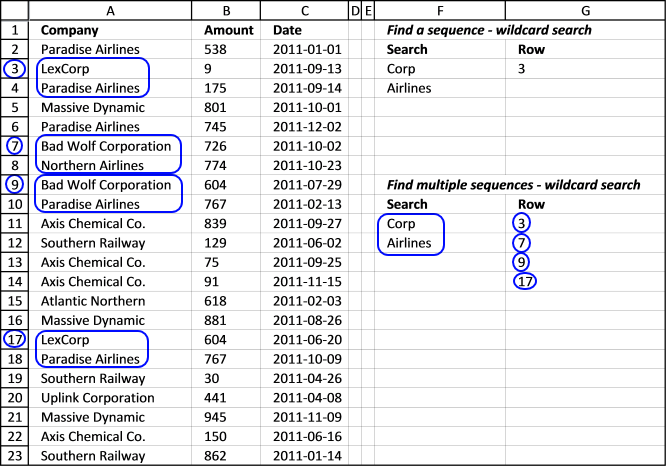This demonstrates how to do wild card searches for multiple sequences. This formula returns the row number of all found sequences in column A. The sequence is in cell F11 and F12.

Array formula in cell G11:

=SMALL(IF(ISNUMBER(FIND(\$F\$11, \$A\$1:\$A\$23)*FIND(\$F\$12, \$A\$2:\$A\$24)), MATCH(ROW(\$A\$1:\$A\$23), ROW(\$A\$1:\$A\$23)), ""), ROW(A1))

If a cell contains "Corp" and the next cell beneath contains "Airlines" the array formula returns the row number.

How to enter an array formula

### Download Excel fileFind-a-sequence-of-values.xlsx

Back to top